# CBSE Class 11 Physics Chapter 4 Revision Notes

Chapter 4: Motion in a Plane Revision Notes

• Motion in two dimensions is defined as motion in a plane, such as projectile motion, circular motion, and so on. For the purpose of analyzing such motion, we will use an origin and two coordinate axes, X and Y.
• Scalar Quantities: are physical quantities that are completely defined by their magnitude or size alone.

Examples: Length, mass, density, velocity, work, and so on.

• Vector Quantities: Physical quantities that have both a magnitude and a direction are known as vector quantities.

Examples: Velocity, displacement, acceleration, force, momentum, torque, and so on are all terms that can be used to describe how fast something is moving.

• Vector Characteristics

(i) Both magnitude and direction are present.

(ii) These do not follow the standard Algebraic rules.

(iii) If the magnitude, direction, or both change, these will change.

(iv) Bold-faced letters or letters with an arrow over them are used to represent these.

• Vector Unit: A unit vector is a vector with the same magnitude and points in a particular direction. Its sole purpose is to specify the direction. A cap (^) is placed over the quantity to represent the unit vector.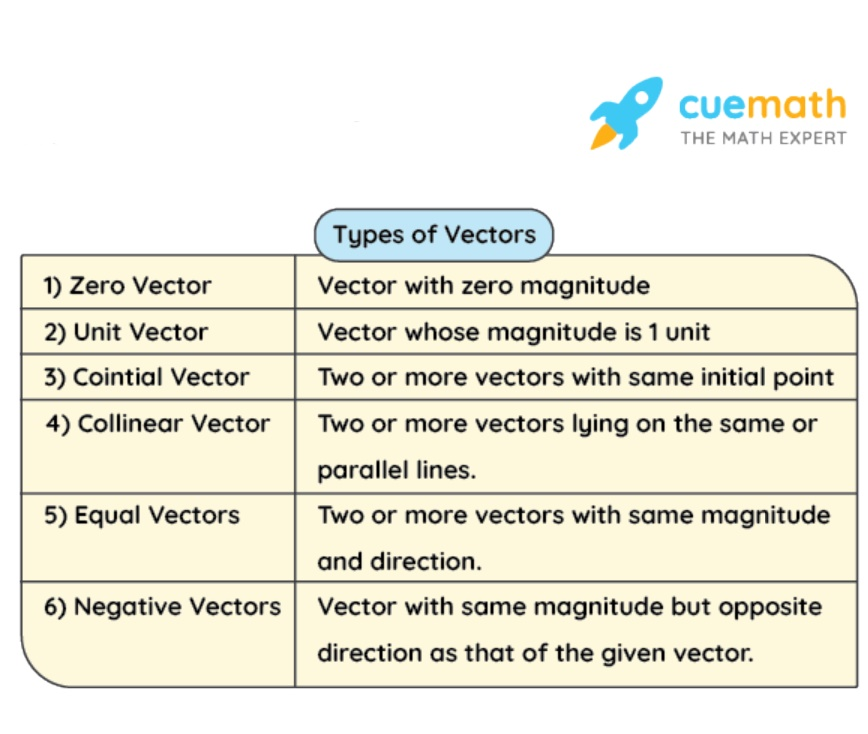Source

• Parallelogram Law of Vector Addition:

If the two adjacent sides of a parallelogram drawn from a point can represent two vectors acting simultaneously at a point in magnitude and direction, then the resultant can be represented completely in magnitude and direction by the diagonal of the parallelogram passing through that point.

• Triangle Law of Vector Addition:

When the magnitude and direction of two vectors are represented by the two sides of a triangle taken in the same order, the resultant of these vectors is represented by the third side of the triangle taken in the opposite order.

• Polygon Law of Vector Addition:

If the sides of a polygon taken in the same order represent a number of vectors in magnitude and direction, the resultant vector is represented in magnitude and direction by the closing side of the polygon taken in the opposite order.

## Projectile Motion in a Plane

• The term “projectile” refers to an object with an initial inclined velocity that then follows a path determined by the gravitational force acting on it as well as the frictional resistance of the air. The trajectory of a projectile is the path it takes.
• Projectile motion equation. The general case of projectile motion is that of an object whose initial velocity u is set at an angle of 8 above (or below) the horizontal. x and y are the horizontal and vertical displacements, respectively.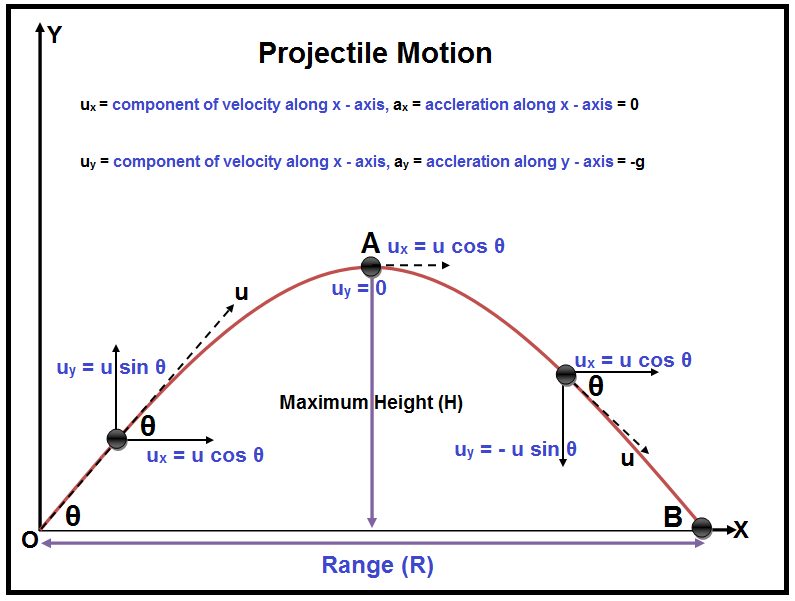Source

• Angular Displacement: The angle traced out by the radius vector at the circular path’s center in a given time is defined as the angular displacement of an object moving around a circular path.

• Angular Velocity: The time rate of change of an object’s angular displacement is defined as its angular velocity in circular motion.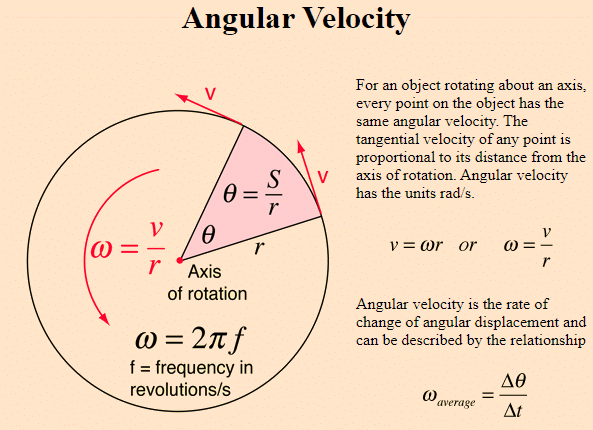Source

• Angular Acceleration:

The time rate of change of an object’s angular velocity is defined as its angular acceleration in circular motion.

• Uniform Circular Motion:

The motion of a body is known as uniform circular motion when it moves in a circular path at a constant speed.

• Time period refers to the amount of time it takes for an object to complete one revolution on its circular path. The frequency of circular motion is defined as the number of revolutions completed per unit time (v). 1 Hertz is the frequency unit (1 Hz).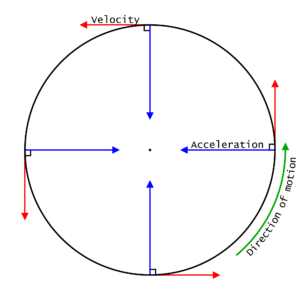Source

• Centripetal Acceleration:

A radially inward acceleration should be maintained continuously to keep a particle in its uniform circular motion. The centripetal acceleration is what it’s called.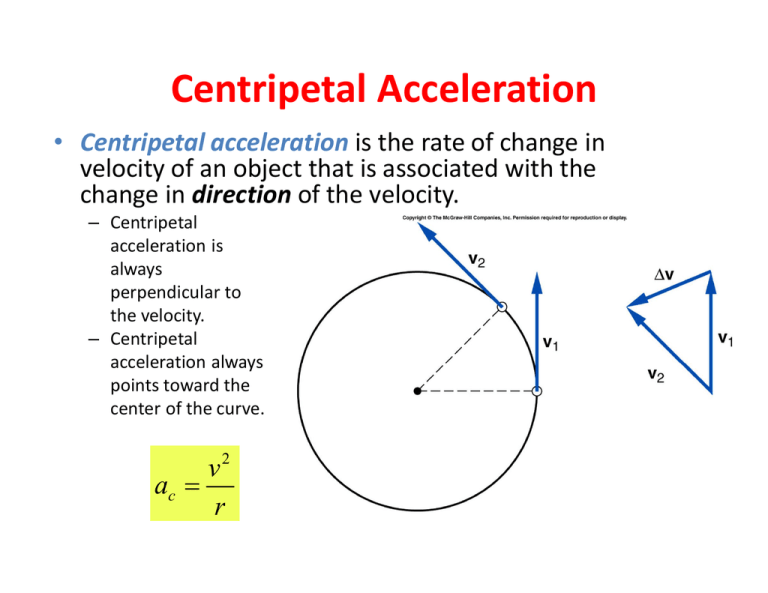Source

]]>